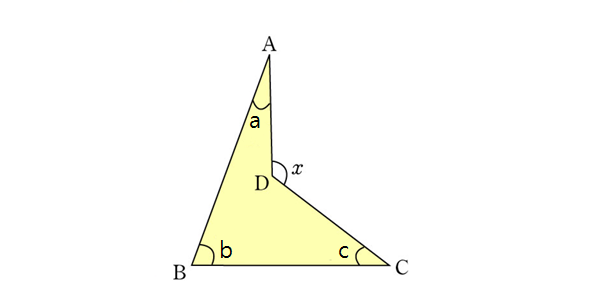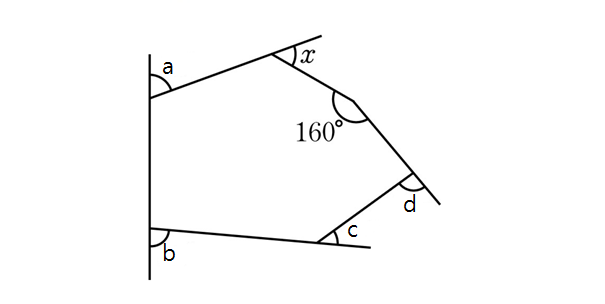Geometry

# Regular Polygons - AnglesIn the above diagram, we are given $\angle a=30^\circ, \angle b=75^\circ, \angle c=40^\circ.$ What is $\angle x?$?In the above diagram, we are given $\angle a=75^\circ, \angle b=90^\circ, \angle c=35^\circ, \angle d=100^\circ.$ What is $\angle x$ in degrees?

Note: The above diagram is not drawn to scale.

A regular polygon has 6 sides. What is the sum (in degrees) of all of its interior angles?

What is the sum of interior angles of a regular $14$-gon?

What is the sum of all exterior angles of a regular polygon with 4 sides?

×